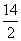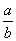Definition Of Divisor

The quantity by which another quantity i.e. the dividend is to be divided is called the Divisor.

If the value of the divisor in an expression is zero, then the expression is undefined.

Examples of Divisor

In the expression= 7, 2 is the divisor.
In the expression= c, b is the divisor.

A. 3
B. 45
C. 5
D. 135